Next: Dirichlet distribution Up: Standard probability distributions Previous: Standard probability distributions   Contents

# Normal distribution

The normal distribution, which is also known as the Gaussian distribution, is ubiquitous in statistics. The averages of identically distributed random variables are approximately normally distributed by the central limit theorem, regardless of their original distribution. This section concentrates on the univariate normal distribution, as the general multivariate distribution is not needed in this thesis.

The probability density of the normal distribution is given by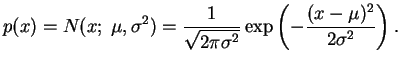(A.1)

The parameters of the distribution directly yield the mean and the variance of the distribution: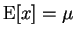,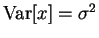.

The multivariate case is very similar: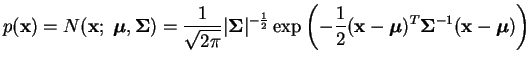(A.2)

where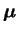is the mean vector and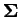the covariance matrix of the distribution. For our purposes it is sufficient to note that when the covariance matrixis diagonal, the multivariate normal distribution reduces to a product of independent univariate normal distributions.

By the definition of the variance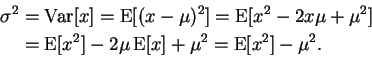(A.3)

This gives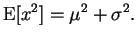(A.4)

The negative differential entropy of the normal distribution can be evaluated simply as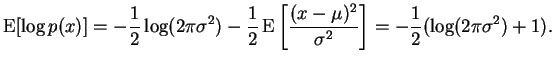(A.5)

Another important expectation for our purposes is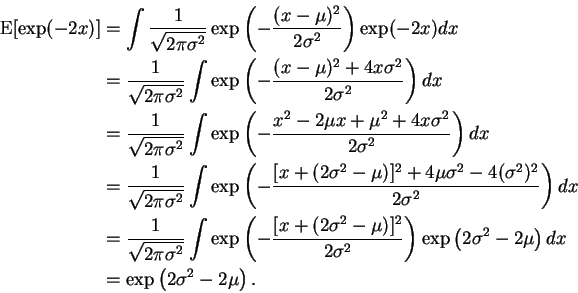(A.6)

A plot of the probability density function of the normal distribution is shown in Figure A.1.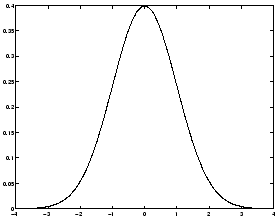Next: Dirichlet distribution Up: Standard probability distributions Previous: Standard probability distributions   Contents
Antti Honkela 2001-05-30